# 图像处理找圆

## 图像处理之霍夫变换圆检测算法

2013-12-10 23:07:36 jia20003 阅读数 45444
• ###### 塑料瓶盖的圆度检测

通过本课程学习，初学者可以熟练使用opencv4 API完成各种常见的图像分割、识别任务。 中高级学习者也一定能有新的体会和收获。 本课程所讲的例子代码来自于实际开发项目，有较高的实用性。

18人学习 刘山
免费试看```	/**
* 霍夫变换处理 - 检测半径大小符合的圆的个数
* 1. 将图像像素从2D空间坐标转换到极坐标空间
* 2. 在极坐标空间中归一化各个点强度，使之在0〜255之间
* 3. 根据极坐标的R值与输入参数(圆的半径)相等，寻找2D空间的像素点
* 4. 对找出的空间像素点赋予结果颜色(红色)
* 5. 返回结果2D空间像素集合
* @return int []
*/
public int[] process() {

// 对于圆的极坐标变换来说，我们需要360度的空间梯度叠加值
acc = new int[width * height];
for (int y = 0; y < height; y++) {
for (int x = 0; x < width; x++) {
acc[y * width + x] = 0;
}
}
int x0, y0;
double t;
for (int x = 0; x < width; x++) {
for (int y = 0; y < height; y++) {

if ((input[y * width + x] & 0xff) == 255) {

for (int theta = 0; theta < 360; theta++) {
t = (theta * 3.14159265) / 180; // 角度值0 ~ 2*PI
x0 = (int) Math.round(x - r * Math.cos(t));
y0 = (int) Math.round(y - r * Math.sin(t));
if (x0 < width && x0 > 0 && y0 < height && y0 > 0) {
acc[x0 + (y0 * width)] += 1;
}
}
}
}
}

// now normalise to 255 and put in format for a pixel array
int max = 0;

// Find max acc value
for (int x = 0; x < width; x++) {
for (int y = 0; y < height; y++) {

if (acc[x + (y * width)] > max) {
max = acc[x + (y * width)];
}
}
}

// 根据最大值，实现极坐标空间的灰度值归一化处理
int value;
for (int x = 0; x < width; x++) {
for (int y = 0; y < height; y++) {
value = (int) (((double) acc[x + (y * width)] / (double) max) * 255.0);
acc[x + (y * width)] = 0xff000000 | (value << 16 | value << 8 | value);
}
}

// 绘制发现的圆
findMaxima();
System.out.println("done");
return output;
}```

```package com.gloomyfish.image.transform.hough;
/***
*
* 传入的图像为二值图像，背景为黑色，目标前景颜色为为白色
* @author gloomyfish
*
*/
public class CircleHough {

private int[] input;
private int[] output;
private int width;
private int height;
private int[] acc;
private int accSize = 1;
private int[] results;
private int r; // 圆周的半径大小

public CircleHough() {
System.out.println("Hough Circle Detection...");
}

public void init(int[] inputIn, int widthIn, int heightIn, int radius) {
width = widthIn;
height = heightIn;
input = new int[width * height];
output = new int[width * height];
input = inputIn;
for (int y = 0; y < height; y++) {
for (int x = 0; x < width; x++) {
output[x + (width * y)] = 0xff000000; //默认图像背景颜色为黑色
}
}
}

public void setCircles(int circles) {
accSize = circles; // 检测的个数
}

/**
* 霍夫变换处理 - 检测半径大小符合的圆的个数
* 1. 将图像像素从2D空间坐标转换到极坐标空间
* 2. 在极坐标空间中归一化各个点强度，使之在0〜255之间
* 3. 根据极坐标的R值与输入参数(圆的半径)相等，寻找2D空间的像素点
* 4. 对找出的空间像素点赋予结果颜色(红色)
* 5. 返回结果2D空间像素集合
* @return int []
*/
public int[] process() {

// 对于圆的极坐标变换来说，我们需要360度的空间梯度叠加值
acc = new int[width * height];
for (int y = 0; y < height; y++) {
for (int x = 0; x < width; x++) {
acc[y * width + x] = 0;
}
}
int x0, y0;
double t;
for (int x = 0; x < width; x++) {
for (int y = 0; y < height; y++) {

if ((input[y * width + x] & 0xff) == 255) {

for (int theta = 0; theta < 360; theta++) {
t = (theta * 3.14159265) / 180; // 角度值0 ~ 2*PI
x0 = (int) Math.round(x - r * Math.cos(t));
y0 = (int) Math.round(y - r * Math.sin(t));
if (x0 < width && x0 > 0 && y0 < height && y0 > 0) {
acc[x0 + (y0 * width)] += 1;
}
}
}
}
}

// now normalise to 255 and put in format for a pixel array
int max = 0;

// Find max acc value
for (int x = 0; x < width; x++) {
for (int y = 0; y < height; y++) {

if (acc[x + (y * width)] > max) {
max = acc[x + (y * width)];
}
}
}

// 根据最大值，实现极坐标空间的灰度值归一化处理
int value;
for (int x = 0; x < width; x++) {
for (int y = 0; y < height; y++) {
value = (int) (((double) acc[x + (y * width)] / (double) max) * 255.0);
acc[x + (y * width)] = 0xff000000 | (value << 16 | value << 8 | value);
}
}

// 绘制发现的圆
findMaxima();
System.out.println("done");
return output;
}

private int[] findMaxima() {
results = new int[accSize * 3];
int[] output = new int[width * height];

// 获取最大的前accSize个值
for (int x = 0; x < width; x++) {
for (int y = 0; y < height; y++) {
int value = (acc[x + (y * width)] & 0xff);

// if its higher than lowest value add it and then sort
if (value > results[(accSize - 1) * 3]) {

// add to bottom of array
results[(accSize - 1) * 3] = value; //像素值
results[(accSize - 1) * 3 + 1] = x; // 坐标X
results[(accSize - 1) * 3 + 2] = y; // 坐标Y

// shift up until its in right place
int i = (accSize - 2) * 3;
while ((i >= 0) && (results[i + 3] > results[i])) {
for (int j = 0; j < 3; j++) {
int temp = results[i + j];
results[i + j] = results[i + 3 + j];
results[i + 3 + j] = temp;
}
i = i - 3;
if (i < 0)
break;
}
}
}
}

// 根据找到的半径R，中心点像素坐标p(x, y)，绘制圆在原图像上
System.out.println("top " + accSize + " matches:");
for (int i = accSize - 1; i >= 0; i--) {
drawCircle(results[i * 3], results[i * 3 + 1], results[i * 3 + 2]);
}
return output;
}

private void setPixel(int value, int xPos, int yPos) {
/// output[(yPos * width) + xPos] = 0xff000000 | (value << 16 | value << 8 | value);
output[(yPos * width) + xPos] = 0xffff0000;
}

// draw circle at x y
private void drawCircle(int pix, int xCenter, int yCenter) {
pix = 250; // 颜色值，默认为白色

int x, y, r2;
r2 = r * r;

// 绘制圆的上下左右四个点

x = 1;
y = (int) (Math.sqrt(r2 - 1) + 0.5);

// 边缘填充算法， 其实可以直接对循环所有像素，计算到做中心点距离来做
// 这个方法是别人写的，发现超赞，超好！
while (x < y) {
setPixel(pix, xCenter + x, yCenter + y);
setPixel(pix, xCenter + x, yCenter - y);
setPixel(pix, xCenter - x, yCenter + y);
setPixel(pix, xCenter - x, yCenter - y);
setPixel(pix, xCenter + y, yCenter + x);
setPixel(pix, xCenter + y, yCenter - x);
setPixel(pix, xCenter - y, yCenter + x);
setPixel(pix, xCenter - y, yCenter - x);
x += 1;
y = (int) (Math.sqrt(r2 - x * x) + 0.5);
}
if (x == y) {
setPixel(pix, xCenter + x, yCenter + y);
setPixel(pix, xCenter + x, yCenter - y);
setPixel(pix, xCenter - x, yCenter + y);
setPixel(pix, xCenter - x, yCenter - y);
}
}

public int[] getAcc() {
return acc;
}

}
```

```package com.gloomyfish.image.transform.hough;

import java.awt.BorderLayout;
import java.awt.Color;
import java.awt.Dimension;
import java.awt.FlowLayout;
import java.awt.Graphics;
import java.awt.Graphics2D;
import java.awt.GridLayout;
import java.awt.event.ActionEvent;
import java.awt.event.ActionListener;
import java.awt.image.BufferedImage;
import java.io.File;

import javax.imageio.ImageIO;
import javax.swing.BorderFactory;
import javax.swing.JButton;
import javax.swing.JFrame;
import javax.swing.JPanel;
import javax.swing.JSlider;
import javax.swing.event.ChangeEvent;
import javax.swing.event.ChangeListener;

public class HoughUI extends JFrame implements ActionListener, ChangeListener {
/**
*
*/
public static final String CMD_LINE = "Line Detection";
public static final String CMD_CIRCLE = "Circle Detection";
private static final long serialVersionUID = 1L;
private BufferedImage sourceImage;
// 	private BufferedImage houghImage;
private BufferedImage resultImage;
private JButton lineBtn;
private JButton circleBtn;
private JSlider numberSlider;
public HoughUI(String imagePath)
{
super("GloomyFish-Image Process Demo");
try{
File file = new File(imagePath);
} catch(Exception e){
e.printStackTrace();
}
initComponent();
}

private void initComponent() {
lineBtn = new JButton(CMD_LINE);
circleBtn = new JButton(CMD_CIRCLE);
numberSlider.setMajorTickSpacing(10);
numberSlider.setMinorTickSpacing(1);
numberSlider.setPaintTicks(true);
numberSlider.setPaintLabels(true);

JPanel sliderPanel = new JPanel();
sliderPanel.setLayout(new GridLayout(1, 2));
sliderPanel.setBorder(BorderFactory.createTitledBorder("Settings:"));
JPanel btnPanel = new JPanel();
btnPanel.setLayout(new FlowLayout(FlowLayout.RIGHT));

JPanel imagePanel = new JPanel(){

private static final long serialVersionUID = 1L;

protected void paintComponent(Graphics g) {
if(sourceImage != null)
{
Graphics2D g2 = (Graphics2D) g;
g2.drawImage(sourceImage, 10, 10, sourceImage.getWidth(), sourceImage.getHeight(),null);
g2.setPaint(Color.BLUE);
g2.drawString("原图", 10, sourceImage.getHeight() + 30);
if(resultImage != null)
{
g2.drawImage(resultImage, resultImage.getWidth() + 20, 10, resultImage.getWidth(), resultImage.getHeight(), null);
g2.drawString("最终结果,红色是检测结果", resultImage.getWidth() + 40, sourceImage.getHeight() + 30);
}
}
}

};
this.getContentPane().setLayout(new BorderLayout());

// setup listener
}

public static void main(String[] args)
{
String filePath = System.getProperty ("user.home") + "/Desktop/" + "zhigang/hough-test.png";
HoughUI frame = new HoughUI(filePath);
// HoughUI frame = new HoughUI("D:\\image-test\\lines.png");
frame.setDefaultCloseOperation(JFrame.EXIT_ON_CLOSE);
frame.setPreferredSize(new Dimension(800, 600));
frame.pack();
frame.setVisible(true);
}

@Override
public void actionPerformed(ActionEvent e) {
if(e.getActionCommand().equals(CMD_LINE))
{
HoughFilter filter = new HoughFilter(HoughFilter.LINE_TYPE);
resultImage = filter.filter(sourceImage, null);
this.repaint();
}
else if(e.getActionCommand().equals(CMD_CIRCLE))
{
HoughFilter filter = new HoughFilter(HoughFilter.CIRCLE_TYPE);
resultImage = filter.filter(sourceImage, null);
// resultImage = filter.getHoughSpaceImage(sourceImage, null);
this.repaint();
}

}

@Override
public void stateChanged(ChangeEvent e) {
// TODO Auto-generated method stub

}
}
```

## 【数字图像处理】利用霍夫变换检测圆形

2016-04-26 16:46:27 jsgaobiao 阅读数 24868
• ###### 塑料瓶盖的圆度检测

通过本课程学习，初学者可以熟练使用opencv4 API完成各种常见的图像分割、识别任务。 中高级学习者也一定能有新的体会和收获。 本课程所讲的例子代码来自于实际开发项目，有较高的实用性。

18人学习 刘山
免费试看

Ø  【作业要求】

Write your own imfindcircles() to simulatematlab function imfindcircles(). The attached images are for testing.

Submit your code, result and report.

Ø  【文件说明】

main.m:

findcircle.m:

Ø  【作业思路】## 利用opencv进行图像处理，提取椭圆圆心处理

2019-03-16 14:32:13 weixin_44540503 阅读数 1750
• ###### 塑料瓶盖的圆度检测

通过本课程学习，初学者可以熟练使用opencv4 API完成各种常见的图像分割、识别任务。 中高级学习者也一定能有新的体会和收获。 本课程所讲的例子代码来自于实际开发项目，有较高的实用性。

18人学习 刘山
免费试看

## 利用opencv进行图像处理，提取椭圆圆心处理

1，二值化处理（threhold()）
2，高斯滤波（GaussianBlur()）
3,轮廓提取（canny算子）
4，寻找闭合轮廓（findContours()）

1，像素点数量过滤（size）
2，面积过滤
3，长宽比过滤

``````#include<iostream>
#include"opencv.hpp"

using namespace std;
using namespace cv;

void drawCross(Mat &img, Point2f point, Scalar color, int size, int thickness /*= 1*/)
{
//绘制横线
line(img, cvPoint(point.x - size / 2, point.y), cvPoint(point.x + size / 2, point.y), color, thickness, 8, 0);
//绘制竖线
line(img, cvPoint(point.x, point.y - size / 2), cvPoint(point.x, point.y + size / 2), color, thickness, 8, 0);
return;
}

//提取单幅图像的特征点
void FeaturePoint(Mat &img)
{
Point2f center; //定义变量
vector<Point2f> ellipsecenterleft;
Mat edges;
threshold(img, img, 80, 255, CV_THRESH_BINARY_INV);
//imshow("threshold", img);
////此函数等待按键，按键盘任意键就返回
//waitKey(0);
GaussianBlur(img, edges, Size(5, 5), 0, 0);
//imshow("GaussianBlur", edges);
////此函数等待按键，按键盘任意键就返回
//waitKey(0);
Canny(edges, edges, 40, 120, 3);
//imshow("Canny", edges);
////此函数等待按键，按键盘任意键就返回
//waitKey(0);
vector<vector<Point> > contours;// 创建容器，存储轮廓
vector<Vec4i> hierarchy;// 寻找轮廓所需参数

findContours(edges, contours, hierarchy, CV_RETR_EXTERNAL, CV_CHAIN_APPROX_NONE);
//cout << "conours size in "<<serialNumber <<" :" << contours.size() << endl;

//if (contours.size() == 1)
for (int i = 0; i < contours.size();i++)
{
RotatedRect m_ellipsetemp;  // fitEllipse返回值的数据类型
if (contours[i].size() <= 200){
continue;
}
if (contourArea(contours[i]) < 100 && contourArea(contours[i]) > 1000)
{
continue;
}
m_ellipsetemp = fitEllipse(contours[i]);  //找到的第一个轮廓，放置到m_ellipsetemp

ellipse(img, m_ellipsetemp, cv::Scalar(255,0,0));   //在图像中绘制椭圆

if (m_ellipsetemp.size.width / m_ellipsetemp.size.height <0.3)
{
continue;
}
center = m_ellipsetemp.center;//读取椭圆中心

drawCross(img, center, Scalar(255,0,0), 30, 2);
cout << center.x << ends << center.y << endl;
}

/*imshow("image", img);
waitKey(0);*/
//return center;//返回椭圆中心坐标

}

int main(int argc, char* argv[])
{
const char* imagename = "pixmap1.png";

//从文件中读入图像

//如果读入图像失败
if (img.empty())
{
fprintf(stderr, "Can not load image %s\n", imagename);
return -1;
}
FeaturePoint(img);
//显示图像
imshow("image", img);

//此函数等待按键，按键盘任意键就返回
waitKey();
return 0;
}
``````

## 图像处理-椭圆检测

2019-05-16 10:11:18 cheng_xing_ 阅读数 1920
• ###### 塑料瓶盖的圆度检测

通过本课程学习，初学者可以熟练使用opencv4 API完成各种常见的图像分割、识别任务。 中高级学习者也一定能有新的体会和收获。 本课程所讲的例子代码来自于实际开发项目，有较高的实用性。

18人学习 刘山
免费试看

# 经典椭圆检测方法

## 投票（聚类）方法

### 随机hough变换椭圆检测算法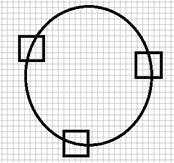1.把边缘检测得到的点收进集合V中，失败计数器f初始值设为0。设定5个阈值，分别是能容忍的失败次数最大值Tf，检测进行时对V中边缘点数量的要求阈值Tem，随机选取的三点之间两两距离最小值Ta，随机选取的第四点到可能椭圆边界距离的最大值Td，以及椭圆残缺比率阈值Tr。
2.np表示集合V中剩余的点的数量，当np小于Tem时或当失败次数f大于Tf时停止检测，算法终止；否则从V中随机取四点，并从V中删除这四点。
3.若用来求解椭圆参数的三个点两两之间距离都大于Ta，拟合出椭圆，计算第四个点到该椭圆边界的距离，若距离小于Td，执行第4步；若不满足两者之一，将这四个点返回到V中，失败次数加一，回到第2步执行。
4.设E为第3步拟合出来的椭圆，初始化满足阈值Td的点的个数num。遍历V中点，计算并判断它们到椭圆E的边界的距离是否小于Td，若是则num=num+1，并将该点从V中除去，直到遍历完成。
5.若num>=Tr*K，其中K为椭圆E的周长，那么跳转到第6步；否则认为椭圆E不是真实的椭圆，将第4步和第2步中删除的num+4个点返回V中，并跳转到第2步。
6.认为椭圆E是一个真实存在的椭圆，f置0，并跳转到第2步。

# 边界聚类算法流程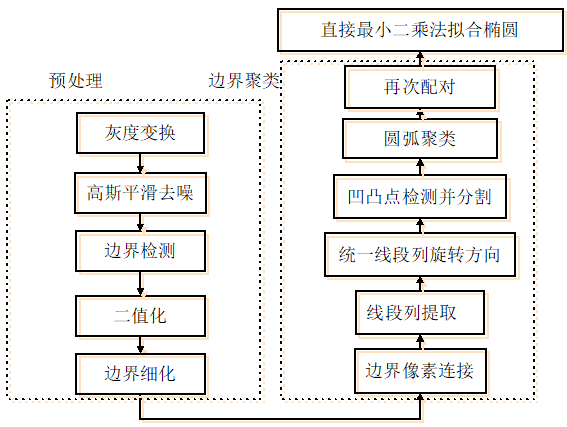## 预处理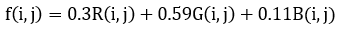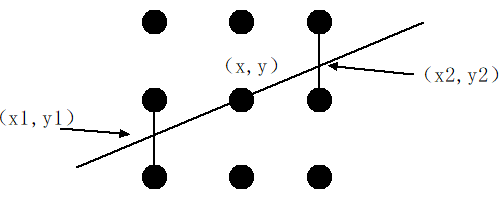## 边界像素连接

1.以8-邻域连通准则从上至下，从左至右扫描二值图像，按连通区域对图像中的像素点聚类。
2.寻找每一个连通域中边界像素中所有的结束点和分叉点（分叉点是三条以上曲线的交点）并存储。
3.以这些结束点和分叉点为结束标志，让每一个连通域中的像素点集合分割为遇到结束点和连接点就断开的小集合。
4.删除这些集合中像素数小于某一阈值的部分。

## 线段列提取

1.取边界像素连接成的第i条有向边界列，判断是否超过边界列总数目total，若不是进行步骤2，若是终止算法。
2.判断该边界列是否已经完成处理，若为否则进行第3步；若是则i=i+1，重新进行步骤2.
3.从其中第三个点开始，计算第一个点到这个点(记为点j)的连线方程，并依次判断第一个点和该点之间的所有点到该连线的距离，若所有距离均小于某一阈值，则j=j+1，重新进行步骤3，否则该有向边界列从该处断开，前面部分只保留第一个点和第j个点，前面j个点构成的连线用第一点和第j点之间的直线连线代替；后面部分仍然记为边界列i，
4.判断步骤3中断开的有向边界列后面部分像素数是否小于某阈值，若是则删除掉，否则不处理。最后跳转到步骤1。

## 线段列旋转方向统一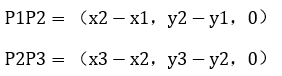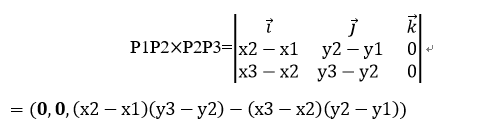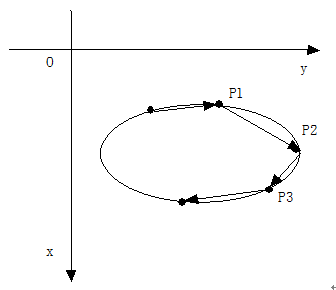## 凹点和角点检测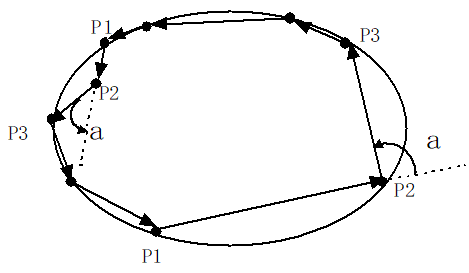## 圆弧聚类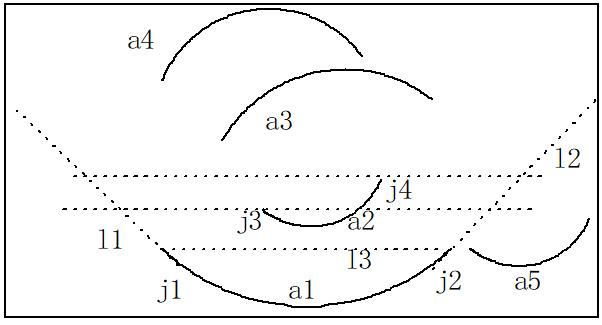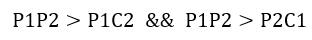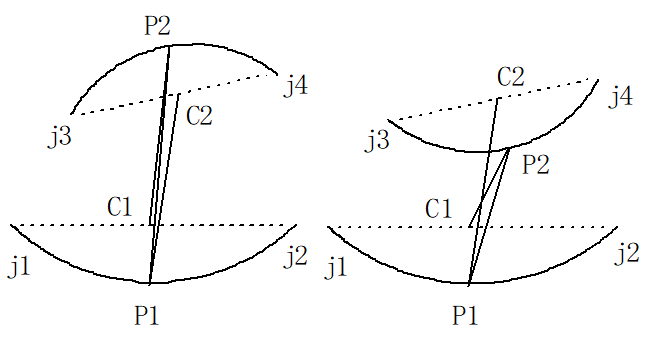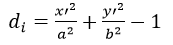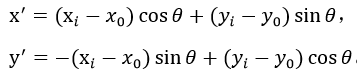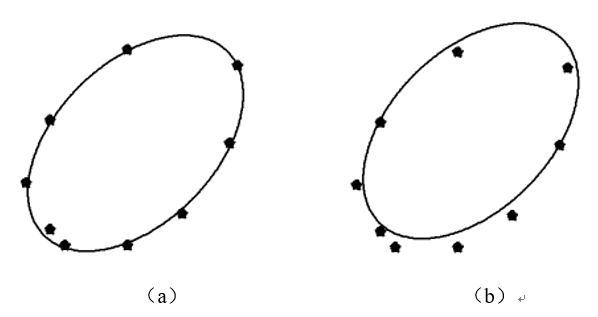# 实验效果

## 边界聚类算法检测结果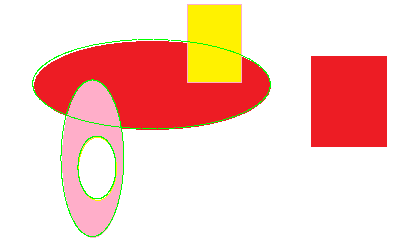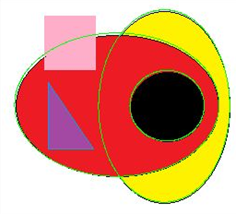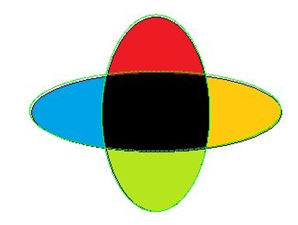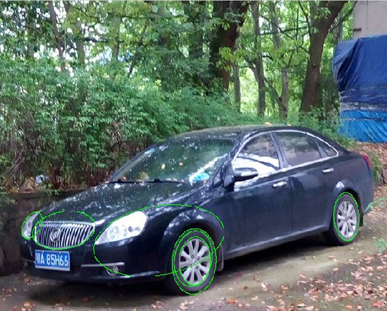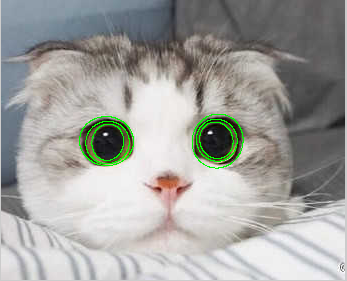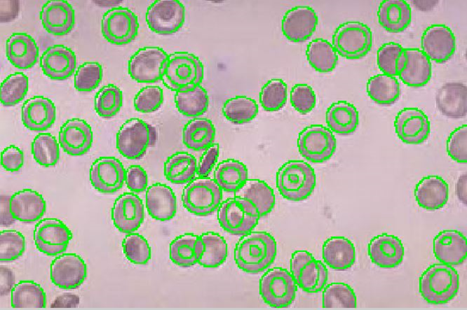## 边界聚类算法和随机霍夫变换算法比较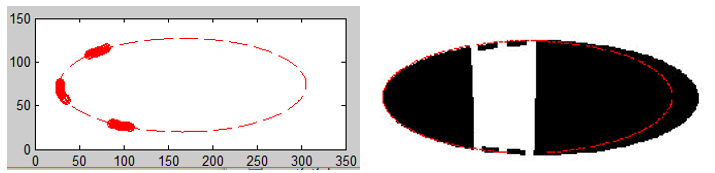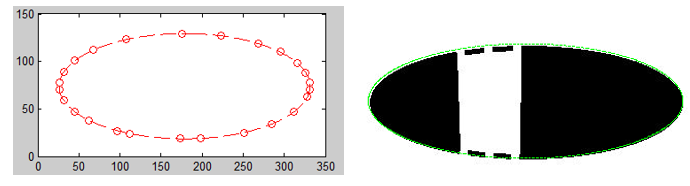## 图像处理中的椭圆拟合（一）

2014-03-30 21:18:14 zhiyuanzhe007 阅读数 9368
• ###### 塑料瓶盖的圆度检测

通过本课程学习，初学者可以熟练使用opencv4 API完成各种常见的图像分割、识别任务。 中高级学习者也一定能有新的体会和收获。 本课程所讲的例子代码来自于实际开发项目，有较高的实用性。

18人学习 刘山
免费试看

图像处理中的椭圆检测用处还是挺多的，找到这里来的同学大多是想用椭圆检测来解决某些实际问题吧，所以我就不做介绍，直奔主题。我研究这块也有一段时间了，也查找了挺多资料，貌似通用的椭圆算法还没有，不排除我孤陋寡闻了。前辈提出的算法适用范围比较有限，这个比较有限是相对直线检测来说的。但直接用Hough变换来找椭圆几乎是不可能的事，在5维空间里做投票，想想都觉得可怕。于是有人想到用随机Hough变换。这是一种很合理的方法，我就是这么做的，不过这种方法有个不足之处，后面会讲到。这里先介绍这方法的流程。

二次曲线的一般方程为：，其中(x,y)为图像的坐标空间，BCDEF是二次曲线的参数。当满足时二次曲线为椭圆。方程中需要求解的参数有5个，在随机Hough变换过程中要至少采集5个点，得到5个方程以求得这5个参数。若方程有解且满足约束条件，则将解加入参数空间进行累积。思路是比较简单的，下面边贴代码边解释（P.S. 代码仅供参考）。

```void FindEllipse(TImage* OrgGrayImg)
{
int ImgHeight = OrgGrayImg->nHeight;
int ImgWidth = OrgGrayImg->nWidth;
unsigned char * Img = OrgGrayImg->pImage; // 输入图像确保是二值图像

srand((unsigned)time(NULL));

int totalPt = 0;// 用于统计样本点的个数

for (i = 0; i < ImgHeight - 0; i++)
{
unsigned char *imgdata = Img + i * ImgWidth;
for (j = 0; j < ImgWidth - 0; j++)
{
if (!imgdata[j])
totalPt ++;
}
}

if (totalPt < 5)
return;

POINT * seq;
seq = new POINT [totalPt];

int count = 0;
for (i = 0; i < ImgHeight; i++)
{
unsigned char *data = Img + i * ImgWidth;
for (j = 0; j < ImgWidth; j++)
{
if (!data[j])
{
seq[count].x = j;
seq[count].y = i;
count ++;
}
}
}

double Para;	// 存放结果（5个参数A,B,C,D,E）的数组
int Angle_V={0};// 椭圆倾斜角参数空间
int *Center_XV = new int[ImgWidth];// 椭圆中心点x坐标参数空间
int *Center_YV = new int[ImgHeight];// 椭圆中心点y坐标参数空间
int *A_axis_V = new int[max(ImgWidth,ImgHeight)/2];// 椭圆长轴参数空间
int *B_axis_V = new int[max(ImgWidth,ImgHeight)/2];// 椭圆短轴参数空间

memset(Center_XV,0,sizeof(int)*ImgWidth);
memset(Center_YV,0,sizeof(int)*ImgHeight);
memset(A_axis_V,0,sizeof(int)*max(ImgWidth,ImgHeight)/2);
memset(B_axis_V,0,sizeof(int)*max(ImgWidth,ImgHeight)/2);

double Theta,X_c,Y_c,A_axis,B_axis;

int loop = 1;// 成功求出参数的迭代次数
int looptop = loop * 1;// 总的迭代次数（也就是控制计算时间的上限，以免陷入无限循环）
while(loop > 0 && looptop > 0)
{
looptop --;
int idx;
for (count = totalPt; count > 0; count--)// 打乱样本点排列的顺序
{
POINT ptrtmp;
idx = rand() % count;

ptrtmp = seq[idx];
seq[idx] = seq[count-1];
seq[count-1] = ptrtmp;
}

double PioMatrix[5*5];
for (i = 0; i < 5; i++)
{
PioMatrix[i*5] = seq[i].x * seq[i].x;
PioMatrix[i*5 + 1] = 2 * seq[i].x * seq[i].y;
PioMatrix[i*5 + 2] = seq[i].y * seq[i].y;
PioMatrix[i*5 + 3] = 2 * seq[i].x;
PioMatrix[i*5 + 4] = 2 * seq[i].y;
}

if (GaussJordanInv(PioMatrix,5) == false)// Gauss-Jordan求逆
continue;
double sum;
for (i = 0; i < 5; i++)
{
sum = 0;
for (j = 0; j < 5; j++)
{
sum +=  -(PioMatrix[i*5 + j]);
}
Para[i] = sum;
}

if (pow(Para,2) - Para * Para > 0)
continue;

if (fabs(Para - Para) < 1e-20)
Theta = 1.570796326;
else if (Para > Para)
Theta = 0.5 * (atan(2.0 * Para / (Para - Para)) + PI);
else
Theta = 0.5 * (atan(2.0 * Para / (Para - Para)));

X_c = (4.0 * Para * Para - 4.0 * Para * Para) / (4.0 * Para * Para - 4.0 * Para * Para);
Y_c = (4.0 * Para * Para - 4.0 * Para * Para) / (4.0 * Para * Para - 4.0 * Para * Para);
A_axis = 2 * (Para * pow(X_c,2) + Para * pow(Y_c,2) + 2 * Para * X_c * Y_c - 1)
/ (Para + Para - sqrt(pow(Para - Para,2) + pow(2.0 * Para,2)));
B_axis = 2 * (Para * pow(X_c,2) + Para * pow(Y_c,2) + 2 * Para * X_c * Y_c - 1)
/ (Para + Para + sqrt(pow(Para - Para,2) + pow(2.0 * Para,2)));

A_axis = sqrt(A_axis);	//长轴
B_axis = sqrt(B_axis);	//短轴

int AngleTmp = (int)(Theta * 180 / PI + 360 + 0.5) % 360;
Angle_V[AngleTmp]++;

if (X_c < 0 || Y_c < 0 || A_axis < 0 || B_axis < 0)
continue;
if (X_c >= ImgWidth || Y_c >= ImgHeight || A_axis > max(ImgWidth,ImgHeight)/2 || B_axis > max(ImgWidth,ImgHeight)/2)
continue;

if (X_c >= 0 && X_c < ImgWidth)
Center_XV[(int)X_c]++;
if (Y_c >= 0 && Y_c < ImgHeight)
Center_YV[(int)Y_c]++;
if (A_axis >= 0 && A_axis < max(ImgWidth,ImgHeight)/2)
A_axis_V[(int)A_axis]++;
if (B_axis >= 0 && B_axis < max(ImgWidth,ImgHeight)/2)
B_axis_V[(int)B_axis]++;
loop--;
}

int Angle,Ai,Bi,Cx,Cy;
//	Angle
int MaxPara = 0;
for (i = 0; i < 360; i++)
{
if (MaxPara < Angle_V[i])
{
MaxPara = Angle_V[i];
Angle = i;
}
}
//	Cy
MaxPara = 0;
for (i = 0; i < ImgHeight; i++)
{
if (MaxPara < Center_YV[i])
{
MaxPara = Center_YV[i];
Cy = i;
}
}
//	Cx
MaxPara = 0;
for (i = 0; i < ImgWidth; i++)
{
if (MaxPara < Center_XV[i])
{
MaxPara = Center_XV[i];
Cx = i;
}
}
//	Ai
MaxPara = 0;
for (i = 0; i < max(ImgWidth,ImgHeight)/2; i++)
{
if (MaxPara < A_axis_V[i])
{
MaxPara = A_axis_V[i];
Ai = i;
}
}
//	Bi
MaxPara = 0;
for (i = 0; i < max(ImgWidth,ImgHeight)/2; i++)
{
if (MaxPara < B_axis_V[i])
{
MaxPara = B_axis_V[i];
Bi = i;
}
}

delete[] Center_XV;
delete[] Center_YV;
delete[] A_axis_V;
delete[] B_axis_V;

double sma = SinMem[Angle];
double cma = CosMem[Angle];
for (int n = 0; n < 360; n++)
{
i = (Bi) * CosMem[360 - n];
j = (Ai) * SinMem[360 - n];

int x,y;
x = (j * cma - i * sma) + Cx;
y = (i * cma + j * sma) + Cy;

Mask[y * ImgWidth + x] = 0;
}
delete[] seq;
}
```当样本点只集中在椭圆的一边时，随机5点的hough变换总会拟合错误，实际应用中往往会发生这样的情况。这是因为公式错了吗？于是我单独提取出五点做测试，即只做一次迭代。测试结果如下图所示。图中实线为实际椭圆，我是用画图工具拖出来的“完美椭圆”，用橡皮擦擦掉一大半部分，最后再做一点旋转。打交叉的是取样的5点，虚线是用这5点代入公式求得的拟合椭圆。可见求得的椭圆穿过了5个点，表明不是求解错了，可就是跟实际的有很大差别。唯一的解释是取样点不在我们想要的椭圆上，也就是说即使是用画图工具拖出来看似完美的椭圆并不完美，这是因为样本点的坐标是整型，精度很低。所以随机5点的hough变换存在很严重的系统误差，当取样点分散在椭圆上下左右时，这种误差会比较小，当集中在某个区域时，误差就会非常大。解决办法就是多采几个点，然后用最小二乘法求解。